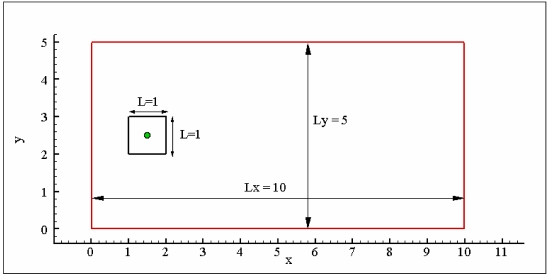## Test 6

2-D Incompressible flow around a moving square inside a rectangular box

(A. Colagrossi)

### Introduction

The flow across a square cylinder is an important test case for the validation of separated flows especially in the turbulent regime. In the past, it has been regularly used to validate Large Eddy Simulation (LES) models.

The test proposed is a tough benchmark for Lagrangian solvers like SPH. The main goal is to prove that the particle solver adopted is able to generate diffuse and convect correctly the unsteady vorticity. Due to the presence of sharp edges the vorticity generated is quite intense.

Even though the geometry is very simple, the flow can be very complex since increasing the Reynolds Number the vorticity decays at lower scales. Three different Reynolds Numbers are here analysed Re=50, 100, 150.

### Flow phenomena

Generation, diffusion and convection of vorticity around a square cylinder. Incompressible Viscous flow around sharp edge. Measure of global loads (pressure and viscous components)

### Geometry

The coordinate system adopted is represented in the sketch below, the red line is the solid boundary of the tank while the black line is the initial position of the square at time t=0. The square is moved rightward for t>0 with a prescribed motion, starting from rest and accelerating to a final steady maximum velocity (see Boundary Conditions).Figure 1. Definition Geometry

### Boundary conditions

This test is characterized only by no-slip solid boundary conditions on all walls of the tank and the square. The file Motion_Body.dat contains four columns which are respectively: time, acceleration, velocity and displacement records for the prescribed motion of the square’s center. These data are normalised to give maximum velocity Umax = 1.0. The test is conducted for three values of Reynolds number (based on L and Umax), 50, 100 and 150.

### Initial conditions

The geometry initial conditions are reported in the “geometry” section. Volume forces (i.e. gravity) are not acting on the fluid. Initially the pressure field is zero everywhere.

### Fluid Properties

The fluid is incompressible and Newtonian.

### Discretisation

The present test has been solved with a Navier-Stokes Finite Difference solver. A regular Cartesian grid has been adopted with 300 points on y-direction and 600 points on the x-direction.

### Results specification

Results to be returned for this test are time histories for the drag coefficient (both pressure and viscous components) for the three Reynolds Numbers 50,100,150. The drag coefficient CD is defined as CD=2*FD/(rho*Umax^2*L*b), where rho is the fluid density, Umax is the maximum velocity of the square, L is the square side, and b the transversal length of the prismatic surface.

### Results format

The time histories of pressure and viscous components of drag coefficients are available in the ASCII files: Force_Re050.dat, Force_Re100.dat, Force_Re150.dat Results should be returned in ASCII files of the following format:

Column 1=time, 2=Cd pressure component, 3= Cd viscous component

The vorticity, pressure and intensity of velocity field contour plots are available on JPG pictures.

### Benchmark results

The present test has been solved with a Navier-Stokes Finite Difference solver, with a Level-Set algorithm to capture fluid/solid interfaces. Grid convergence has been tested for all the three Reynolds numbers using three different grid resolutions: 600×300; 400×200; 267×133 (see e.g. images below)

The time histories of pressure and viscous components of drag coefficients are available in the ASCII files: Force_Re050.dat, Force_Re100.dat, Force_Re150.dat.

Attention: drag coefficient time records present some small amplitude and high frequency oscillations which are due to numerical reasons and have not to be taken into account for the comparison. The following field data are available as jpg images:

### SPH Publications using this Case

• Cherfils J-M, Blonce L, Pinon G, Rivoalen E. "IB-SPH simulations of wave-body interactions", Proc. 5th International SPHERIC Workshop, Editor B.D. Rogers. pp 83-89. 23-25 June 2010.

• Ferrand M, Laurence D R P, Rogers B D, Violeau D. "Improved time scheme integration approach for dealing with semi analytical boundary conditions in SPARTACUS2D", Proc. 5th International SPHERIC Workshop, Editor B.D. Rogers. pp 98-105. 23-25 June 2010.

If you have published results for this case, please email the webmaster to have your papers added.

### References

G. Colicchio, M. Greco, O.M. Faltinsen, 2006, Fluid-body interaction on a Cartesian grid: dedicated studies for a CFD validation Proc. 21st International Workshop on Water Waves and Floating Bodies, IWWWFB (Loughborough, UK)